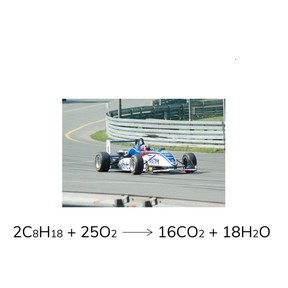Chemical equations

# Chemical equations

I can read and interpret chemical equations.8,000 schools use Gynzy92,000 teachers use Gynzy1,600,000 students use Gynzy

## General

In this lesson, students will learn about the symbols in chemical equations and how to determine if they are balanced.

NGSS:
MS-PS1-1
MS-PS1-5

## Learning objective

Students will be able to read and interpret chemical equations.

## Introduction

Students will answer questions to review the periodic table. They will also look at equations, such as 3a + 4b = 25 and y = mx + b, and write down some common symbols they see. They will talk about how math symbols, like = and +, are used in chemical equations.

## Instruction

Introduce chemical equations, reactants, and products. Students will find the reactants and products in 2HCl → H₂ + Cl₂. Discuss the common symbols seen in chemical equations, including, chemical symbols, plus (+), and yield (→). Students will recall some chemical symbols prompted in the lesson.

Next, explain the purpose of the coefficient, and the difference between a coefficient and a subscript. Explain that products and reactants are balanced in chemical equations. Students will determine whether the equation 2H₂ + N₂ → 2NH₃ is balanced.

## Quiz

Students will respond to ten true/false and multiple-choice questions.

## Closing

Students will recall what they learned in the lesson and complete an exit slip.

### The online teaching platform for interactive whiteboards and displays in schools

• Save time building lessons

• Manage the classroom more efficiently

• Increase student engagement